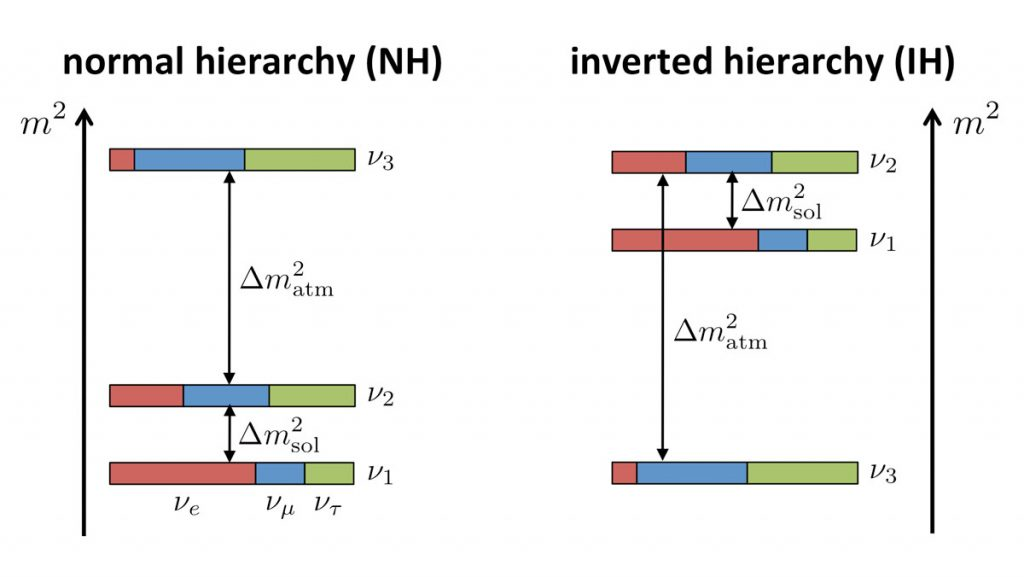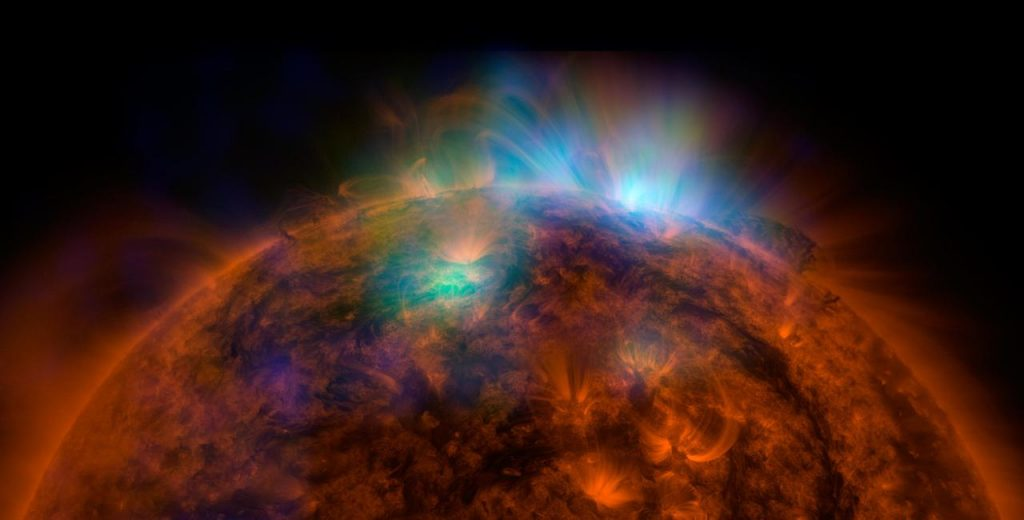# Which neutrino is the lightest?

The mystery of neutrino mass doesn’t stop at how much they weigh—it also extends to which one is the lightest.

We know there are three flavors of neutrinos: electron, muon, and tau neutrinos. But there are also three different mass neutrinos: ν1, ν2, and ν3, alternately referred to as mass 1, mass 2, and mass 3. If each flavor of neutrino matched up with one specific mass, then life would be simple—but boring. Neutrinos are tricky, and it turns out that the mass and flavor neutrinos do not overlap perfectly.

Instead, quantum mechanics comes into play. Each neutrino of a specific flavor is actually a combination of neutrinos of different masses. As a result, each neutrino of a specific mass has a certain chance of interacting as a particular flavor. For example, ν1 is very likely to interact as an electron neutrino.

The important thing to know for this mystery is that scientists don’t know whether ν3 is much heavier or much lighter than the other two mass state neutrinos. And if it is the lightest, it would be a big surprise!

Neutrino physicists often talk about two scenarios for how neutrino mass might shake out. (This ordering of neutrino masses is sometimes given the term “hierarchy.”)

In the “normal mass ordering,” ν1 is the lightest, ν2 is the middle-weight, and the ν3 is the heaviest. This is called normal because it mirrors the masses of the particles that are associated with neutrinos. The electron is much lighter than the muon and tau particles, so it seems normal that the mass neutrino that usually shows up as an electron neutrino would be lighter than the other mass neutrinos. Put another way, the electron neutrino is mostly made up of the neutrino mass state that is the lightest of the mass states.

The other option is the “inverted mass ordering,” where the ν3 neutrino is the lightest. The ν1 neutrinos aren’t the lightest of all—meaning electron neutrinos aren’t made of the lightest mass state, as one would expect. Put another way, the electron neutrino is mostly made of one of the heavier neutrino mass states. Talk about flipping particles on their heads!

Scientists can check whether neutrinos come in the normal or inverted mass ordering with experiments that look at how neutrinos change over long distances. If things are “normal,” certain neutrino oscillations (changes between flavors) should happen at a higher rate than in the inverted world.

Neutrinos are observed in the three flavors that correspond to the leptons (electron, muon, and tau) that are produced when the neutrinos interact. In the simplest explanation for neutrino flavor change, the three neutrino flavors are quantum mechanical combinations of three neutrino mass states. This means that neutrinos travel as a combination of the three mass states rather than as a single, static flavor.

Each of those mass states has a probability of interacting as each of the flavors.

• One mass state (ν1) will interact as an electron neutrino roughly two-thirds of the time, and as a muon or tau neutrino one-third of the time.
• Another mass state (ν2) will interact as any of the three flavors roughly one-third of the time.
• The final mass state (ν3) will interact as a muon or tau neutrino roughly 45 percent of the time each, and an electron neutrino roughly 10 percent of the time.

The probability that the neutrino flavor at the point of interaction is different from the flavor at the point of creation depends on the speeds at which the three mass neutrinos move. This fact tells scientists both that neutrinos have mass and that those masses are different, because if neutrinos were massless, they would all travel at the speed of light.Two potential mass orderings of neutrinos are the normal ordering (normal hierarchy) and the inverted ordering (inverted hierarchy). Credit: JUNO Collaboration / JGU-Mainz

Scientists have figured out a few things about the mass ordering of neutrinos in experiments.

By observing how electron neutrinos produced in the sun change into muon and tau neutrinos, they learned that ν1 and ν2 have very similar masses. Mass 2 is slightly heavier than mass 1, and the difference in the squares of the masses is less than one part in 10,000. Thus, they know the order of these two neutrino mass states.

How did scientists figure that out? Neutrinos produced in the sun are electron neutrinos, and scientists know that electron neutrinos are most likely to be ν1 (see above). Scientists also know that they’re likely to interact as electron neutrinos. As they propagate outward from the core of the sun, the neutrinos gain an “effective mass” due to the presence of electrons. This interaction with the electrons in the sun transitions the neutrinos into the second mass state, which interact equally as electron, muon, or tau neutrinos. Because scientists saw only one-third of the electron neutrinos expected to be produced in the sun, they deduced that the mass state observed in detectors is heavier than the state produced in our star.The sun emits electron neutrinos, though this picture taken by NASA’s Solar Dynamics Observatory shows X-rays streaming off instead. Credit: NASA/JPL-Caltech/GSFC

Researchers also know a bit about how ν3 relates to ν1 and ν2 thanks to atmospheric neutrinos, which are produced when charged particles interact in Earth’s upper atmosphere. These neutrinos are produced primarily as muon neutrinos. Observations of the number of these muon neutrinos interacting in detectors around the world have shown that the differences in the squares of the masses between the third mass state and the other two mass states is much larger than the difference between the first and second mass states. In this case, the difference is about one part in 400. Or, to say it another way, mass 3 is very different from mass 1 and mass 2.

What scientists have not yet determined is whether the mass 3 neutrino is heavier or lighter than the other two. To figure it out, they are performing experiments where neutrinos are created at known energies and then allowed to propagate through enough of Earth, producing a similar effect to what happens when electron neutrinos travel through the sun. If the third mass state is lighter than the others, then the oscillation probability will be lower than expected when the neutrinos travel through vacuum, while if it is heavier, the oscillation probability will be higher than expected in vacuum. The opposite relationship is true for antineutrinos, and experiments compare the differences between the two to look for differences between matter and antimatter.

Physicists are not yet sure what the ordering of the neutrino masses tells us about the universe. There are many possible Grand Unified Theories, which seek to explain particle interactions as different manifestations of a single force, that predict the mass 3 neutrino is heavier than the other two. On the other hand, if the third mass state were lighter, a host of other theories about the nature of matter would become possible. In the end, scientists have to measure the ordering of the masses first, before they can use that information as a piece of the puzzle to learn more about the nature of matter and the universe.